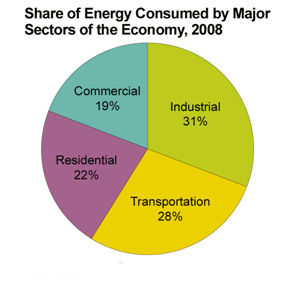Motor controls
Home » Blog » Motor controls » Measuring inductance in per coils of switched reluctance motors

# Measuring inductance in per coils of switched reluctance motors

Fundamentally, inductance is the proportionality constant relating flux linkage to current, i.e. lambda = L * i, where lamda is flux linkage, L is inductance, and i is current. Inductance is a property of the geometry. One can write L = N^2 *R, where N = number of turns in the coil and R = the magnetic reluctance "seen" by the coil. So here are a couple of comments. The reluctance varies in an SR motor as the rotor turns, so you will have to take measurements at several positions. If the current is small, L is a constant. If i is large then a small increase in i produces a small increase in lambda, which is smaller than when the current is small. So, you'll need to decide on the level of current; or, you'll need to make measurements at several levels of current. For example, at the rated value of current and half the rated value, etc. Now flux linkage is the product of turns and the effective flux through the coil, lamda = N * phi, where phi is the effective flux through the coil. In the case, you have two coils in series where the coils are inside a motor. You only have two leads, one each to the two coils in series. So, you will need to disassemble the motor and tap into a wire that connects the two coils in series. One way to find the flux linkage is the following. Apply a step of voltage to one of your coils. Take traces of the voltage and current. Then apply the formula v = R' * i + d(lamdda)/dt, where v is the applied voltage to the coil, R' is the coil resistance (you'll have to measure this), and i is the current. Then, integrate to find lamda: d(lamda) = int(v - R'*i)dt. You'll have to do this to both coils.

As you know the inductance of SRM depends on two parameters: 1.coil current 2.rotor position .it means that you have a lot of possible situation that each situation has particular value of inductance .if you want to measure inductance at particular position, I think you should excite one phase with ac supply and use circuit equations (kvl) to find inductance. if you use a dc supply you should measure the flux and it's hard to do.

Calculate (9 * 9) =

You may also like:

It will cause a series problems during AC drive operation in various environmental conditions, take an example as: when failure occurs, AC drives protective function is activated, and the AC drive tripped ...
Induction motor is inductive load, the current lags the voltage, most electrical appliances are the same. In order to improve the power factor we need to use capacitive load for compensation, parallel ...
Remote diagnostic is a must now a days. All CNC machines must be able to undergo remote access to undergo diagnostic and it must be two way. The problem mostly with remote diagnostic is it has to be two way ...
I would like to share these tips with everybody. A current mode controlled flyback converter always becomes unstable at low load due to the unavoidable leading edge current spike. It is not normally dangerous ...
Always the top brands will be the most popular and over many years it is my opinion that this is because of their marketing strategy, history, reputation and worldwide acceptance more than any other reasons. ...Gozuk Blog: all about electric motor control & drives industries development in energy saving applications.

Featured

Like pumps, fans consume significant electrical energy while serving several applications. In many plants, the VFDs (variable ...A frequency inverter controls AC motor speed. The frequency inverter converts the fixed supply frequency (60 Hz) to a ... Motor starter (also known as soft starter, motor soft starter) is a electronic device integrates soft start, soft stop, ... Soft starter allows the output voltage decreases gradually to achieve soft stop, in order to protect the equipment. Such as the ... Soft Starter reduces electric motor starting current to 2-4 times during motor start up, reduces the impact to power grid during ...

In Discussion